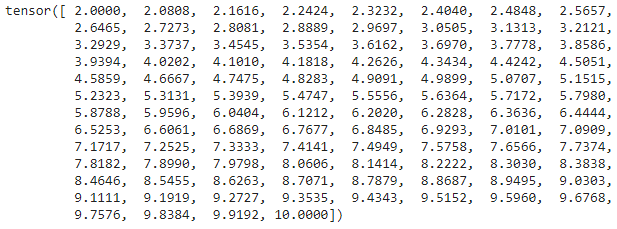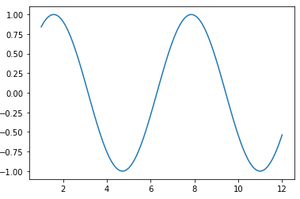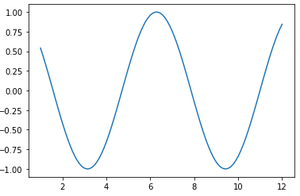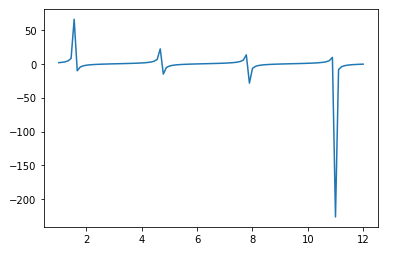Open In App

# Vector Operations in Pytorch

In this article, we are going to discuss vector operations in PyTorch. Vectors are a one-dimensional tensor, which is used to manipulate the data. Vector operations are of different types such as mathematical operation, dot product, and linspace.

PyTorch is an optimized tensor library majorly used for Deep Learning applications using GPUs and CPUs. It is one of the widely used Machine learning libraries, others being TensorFlow and Keras.

We can create a vector by using the torch.tensor() function

Syntax:

torch.tensor([value1,value2,.value n])

where values are the input values that take input as a list

Example:

## Python3

 `# importing pytorch module``import` `torch` `# create an vector``A ``=` `torch.tensor([``7058``, ``7059``, ``7060``, ``7061``, ``7062``])` `# display``print``(A)`

Output:

tensor([7058, 7059, 7060, 7061, 7062])

Now let us discuss each vector operation supported under tensor.

### Arithmetic operations

The procedure is extremely simple, just create two vectors and perform operations on them like you are performing them on two regular variables.

Example:

## Python3

 `# importing pytorch module``import` `torch` `# create an vector A``A ``=` `torch.tensor([``58``, ``59``, ``60``, ``61``, ``62``])` `# create an vector B``B ``=` `torch.tensor([``100``, ``120``, ``140``, ``160``, ``180``])` `# add two vectors``print``(``"Addition of two vectors:"``, A``+``B)` `# subtract two vectors``print``(``"subtraction of two vectors:"``, A``-``B)` `# multiply two vectors``print``(``"multiplication of two vectors:"``, A``*``B)` `# multiply two vectors``print``(``"multiplication of two vectors:"``, A``*``B)` `# divide two vectors``print``(``"division of two vectors:"``, A``/``B)` `# floor divide two vectors``print``(``"floor division of two vectors:"``, A``/``/``B)` `# modulus of two vectors``print``(``"modulus operation of two vectors:"``, A ``%` `B)` `# power of two vectors``print``(``"power operation of two vectors:"``, A``*``*``B)`

Output:

Addition of two vectors: tensor([158, 179, 200, 221, 242])

subtraction of two vectors: tensor([ -42,  -61,  -80,  -99, -118])

multiplication of two vectors: tensor([ 5800,  7080,  8400,  9760, 11160])

multiplication of two vectors: tensor([ 5800,  7080,  8400,  9760, 11160])

division of two vectors: tensor([0.5800, 0.4917, 0.4286, 0.3812, 0.3444])

floor division of two vectors: tensor([0, 0, 0, 0, 0])

modulus operation of two vectors: tensor([58, 59, 60, 61, 62])

power operation of two vectors: tensor([  0, -4166911448072485343,  0,8747520307384418433,  0])

### Unary operations

It is similar to arithmetic operations except that the other vector part is replaced by a constant.

Example:

## Python3

 `# importing pytorch module``import` `torch` `# create an vector A``A ``=` `torch.tensor([``58``, ``59``, ``60``, ``61``, ``62``])` `# divide vector by 2``print``(A``/``2``)` `# multiply vector by 2``print``(A``*``2``)` `# subtract  vector by 2``print``(A``-``2``)`

Output:

tensor([29.0000, 29.5000, 30.0000, 30.5000, 31.0000])

tensor([116, 118, 120, 122, 124])

tensor([56, 57, 58, 59, 60])

### Dot product

dot() is used to get the dot product. The vectors in consideration just need to be passed to it.

Syntax:

torch.dot(vector1,vector2)

Example:

## Python3

 `# importing pytorch module``import` `torch` `# create an vector A``A ``=` `torch.tensor([``58``, ``59``, ``60``, ``61``, ``62``])` `# create an vector B``B ``=` `torch.tensor([``8``, ``9``, ``6``, ``1``, ``2``])` `# dot product of the two vectors``print``(torch.dot(A, B))`

Output:

tensor(1540)

### Linspace function

linspace is used to arrange data linearly in the given space. It is available in the torch package and using linspace() function with values for start and end are enough.

Syntax:

torch.linspace(start,end)

where start is the starting value and end is the ending value.

Example

## Python3

 `# importing pytorch module``import` `torch` `# arrange the elements from 2 to 10``print``(torch.linspace(``2``, ``10``))`

Output:### Plotting a function on the two-dimensional coordinate system

The linspace function is used to plot a function on two-dimensional coordinate systems. For the x-axis, we create a land space from 0 to 10 in an interval of 2.5, and Y will be the function of each x value.

Example 1: sin function

## Python3

 `#import pytorch``import` `torch` `#import numpy``import` `numpy as np` `#import matplotlib``import` `matplotlib.pyplot as plt` `# create lin space from 1 to 12``x ``=` `torch.linspace(``1``, ``12``)` `# sin function``y ``=` `torch.sin(x)` `# plot``plt.plot(x.numpy(), y.numpy())` `# display``plt.show()`

Output:Example 2: cos function

## Python3

 `#import pytorch``import` `torch` `#import numpy``import` `numpy as np` `#import matplotlib``import` `matplotlib.pyplot as plt` `# create lin space from 1 to 12``x ``=` `torch.linspace(``1``, ``12``)` `# cos function``y ``=` `torch.cos(x)` `# plot``plt.plot(x.numpy(), y.numpy())` `# display``plt.show()`

Output:Example 3: tan() function

## Python3

 `#import pytorch``import` `torch` `#import numpy``import` `numpy as np` `#import matplotlib``import` `matplotlib.pyplot as plt` `# create line space from 1 to 12``x ``=` `torch.linspace(``1``, ``12``)` `# tan function``y ``=` `torch.tan(x)` `# plot``plt.plot(x.numpy(), y.numpy())` `# display``plt.show()`

Output: